# Exercise E7.5 Triangles NCERT Solutions Class 9

## Chapter 7 Ex.7.5 Question 1

$$ABC$$ is a triangle. Locate a point in the interior of $$ΔABC$$ which is equidistant from all the vertices of $$ΔABC.$$

### Solution

Steps:

Circumcentre of a triangle is always equidistant from all the vertices of that triangle.

Circumcentre is the point where perpendicular bisectors of all the sides of the triangle meet together.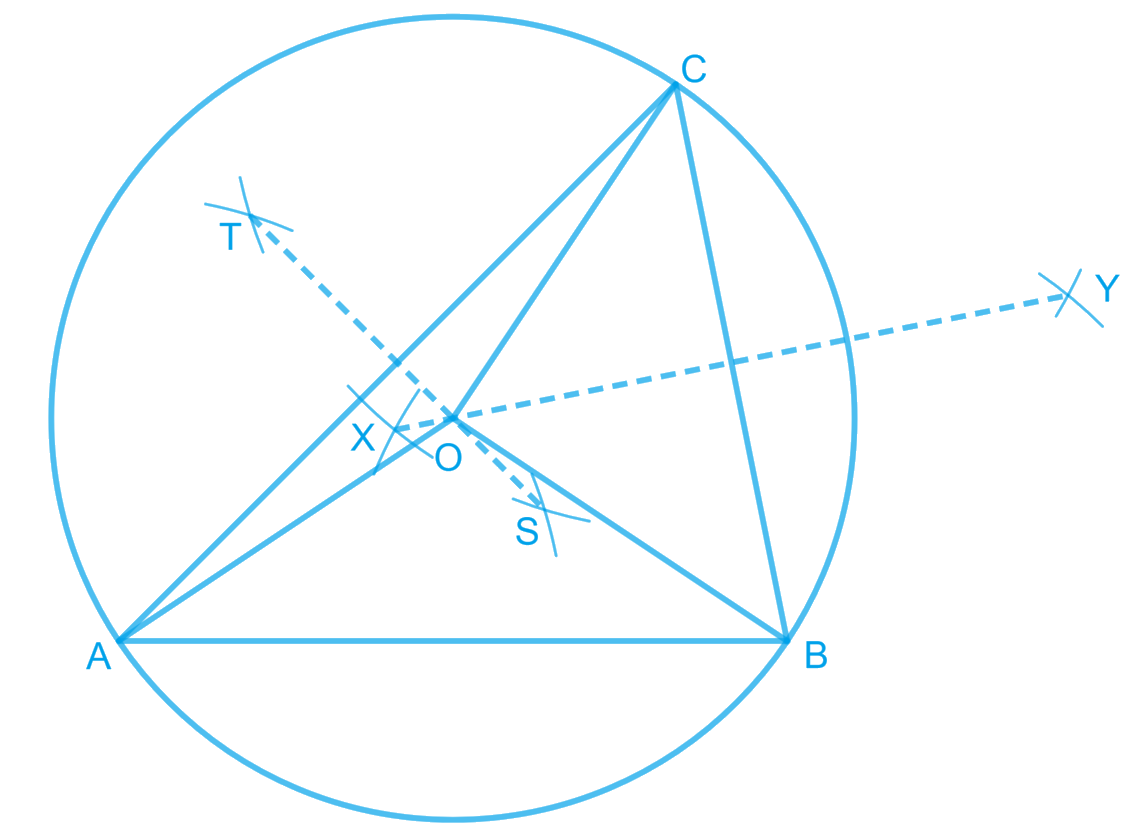In $$\Delta ABC$$, we can find the circumcentre by drawing the perpendicular bisectors of sides $$AB, BC,$$ and $$CA$$ of this triangle. $$O$$ is the point where these bisectors are meeting together. Therefore, $$O$$ is the point which is equidistant from all the vertices of $$\Delta ABC$$.

## Chapter 7 Ex.7.5 Question 2

In a triangle locate a point in its interior which is equidistant from all the sides of the triangle.

### Solution

Steps:

The point which is equidistant from all the sides of a triangle is called the incentre of the triangle. Incentre of a triangle is the intersection point of the angular bisectors of the interior angles of that triangle.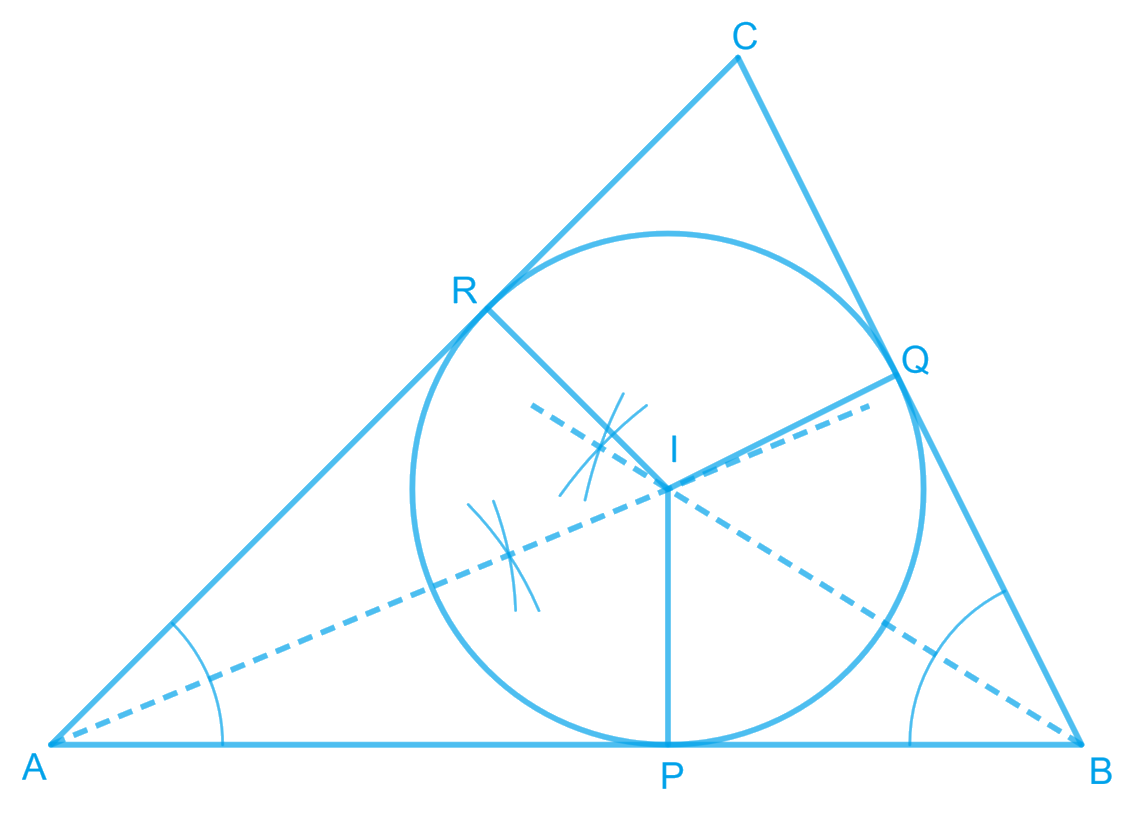Here, in $$\Delta ABC$$, we can find the incentre of this triangle by drawing the angular bisectors of the interior angles of this triangle. I is the point where these angle bisectors are intersecting each other. Therefore, I is the point which is equidistant from all the sides of $$\Delta ABC$$.

## Chapter 7 Ex.7.5 Question 3

In a huge park people are concentrated at three points (see the given figure)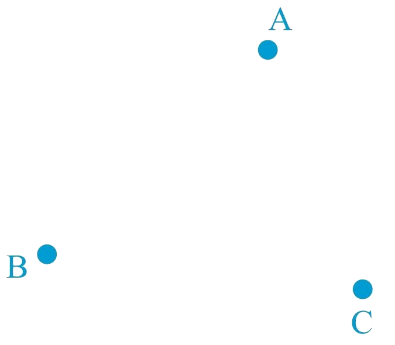### Solution

A: where there are different slides and swings for children,

B: near which a man-made lake is situated,

C: which is near to a large parking and exit.

Where should an ice-cream parlour be set up so that maximum number of persons can approach it?

(Hint: The parlor should be equidistant from $$A, B$$ and $$C$$)

Steps:

Maximum number of persons can approach the ice-cream parlour if it is equidistant from $$A, B$$ and $$C$$. Now, $$A, B$$ and $$C$$ form a triangle. In a triangle, the circumcentre is the only point that is equidistant from its vertices. So, the ice-cream parlour should be set up at the circumcentre $$O$$ of $$\Delta ABC$$.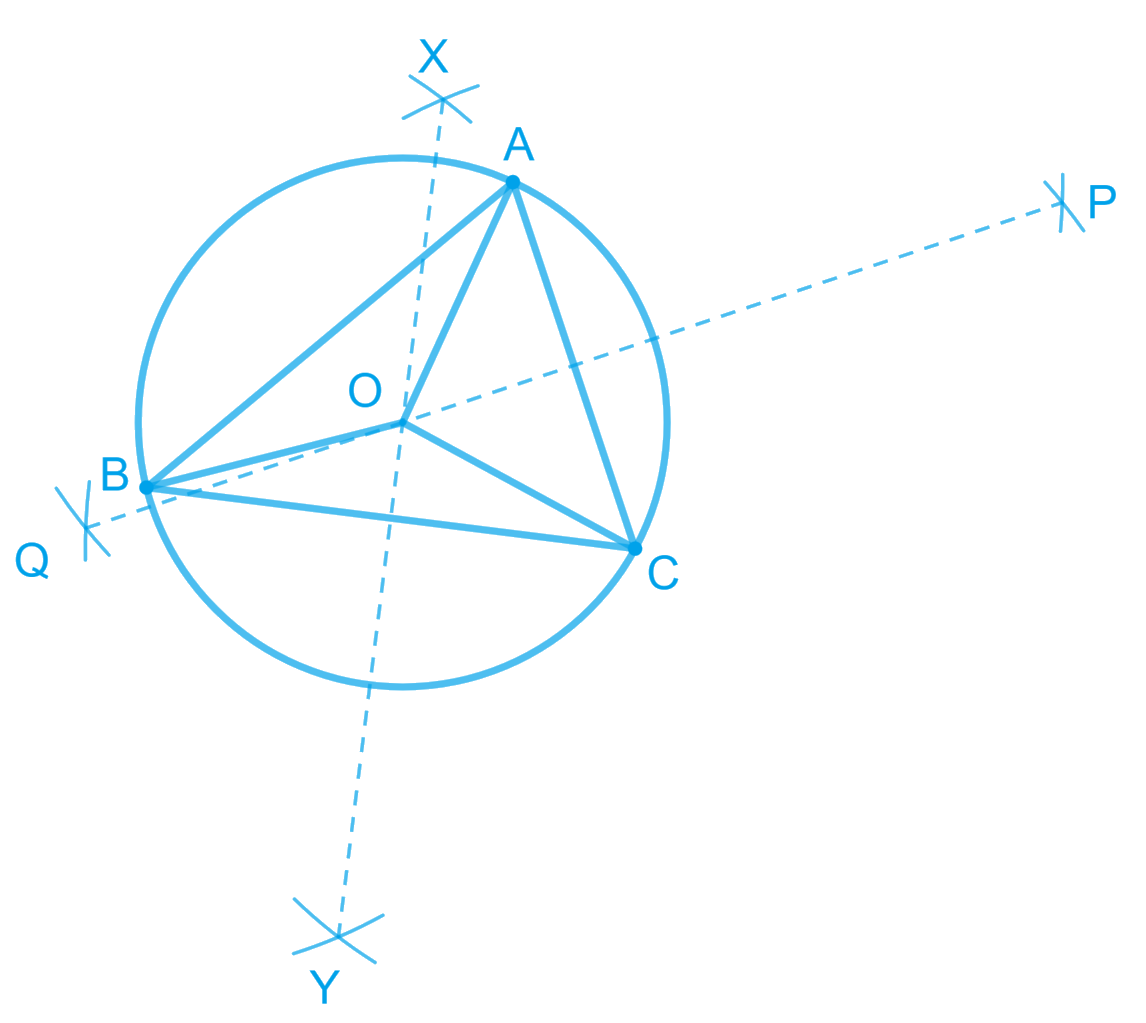In this situation, maximum number of persons can approach it. We can find circumcentre $$O$$ of this triangle by drawing perpendicular bisectors of the sides of this triangle.

## Chapter 7 Ex.7.5 Question 4

Complete the hexagonal and star shaped rangolies (see the given figures) by filling them with as many equilateral triangles of side $$1\,\rm cm$$ as you can. Count the number of triangles in each case. Which has more triangles?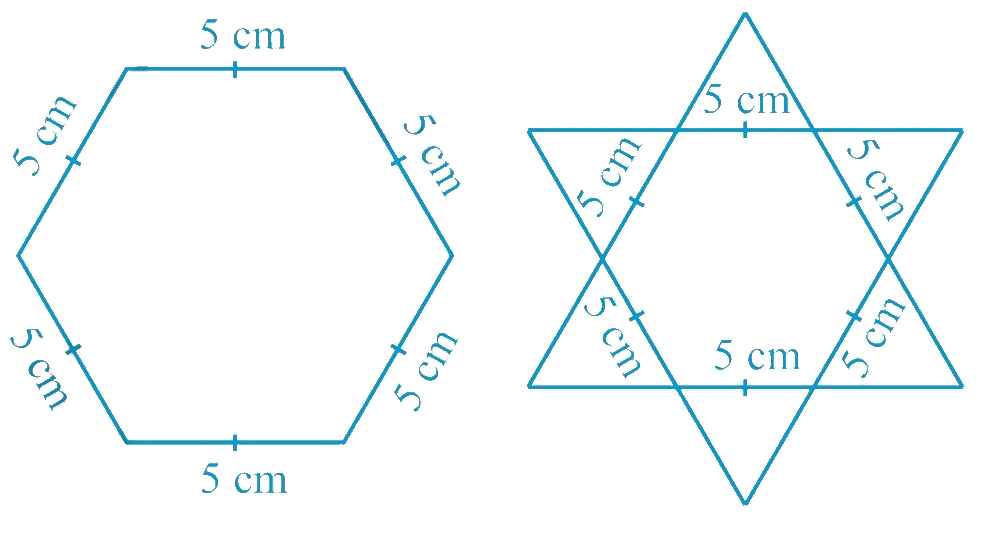### Solution

Steps:

It can be observed that hexagonal-shaped rangoli has 6 equilateral triangles in it.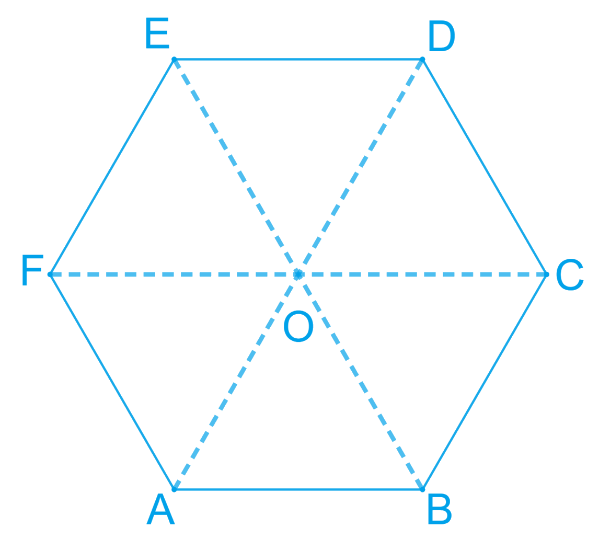Area of an equilateral Triangle=$$\frac { \sqrt { 3 } } { 4 } ( \text { side } ) ^ { 2 }$$

\begin{align}\text{Area of } \Delta OAB &= \frac { \sqrt { 3 } } { 4 } ( \text {side} )^{2}\\&= \frac { \sqrt { 3 } } { 4 } ( 25 ) \\ &= \frac { 25 \sqrt { 3 } } { 4 }\; cm ^ { 2 } \end{align}

Area of hexagonal-shaped Rangoli

\begin{align} &= 6 \times \frac { 25 \sqrt { 3 } } { 4 } \\ &= \frac { 75 \sqrt { 3 } } { 2 }\;cm ^ { 2 } \end{align}

Area of equilateral triangle having its side as $$1\,\rm cm$$

\begin{align} & = \frac { \sqrt { 3 } } { 4 } ( 1 ) ^ { 2 } \\ & = \frac { \sqrt { 3 } } { 4 } \; cm ^ { 2 } \end{align}

Number of equilateral triangles of 1 cm side that can be filled in this hexagonal-shaped Rangoli

\begin{align} & = \left( \frac { \frac { 75 \sqrt { 3 } } { 2 } } { \frac { \sqrt { 3 } } { 4 } } \right)\\ & =150 \end{align}

Star-shaped rangoli has $$12$$ equilateral triangles of side $$5\,\rm cm$$ in it.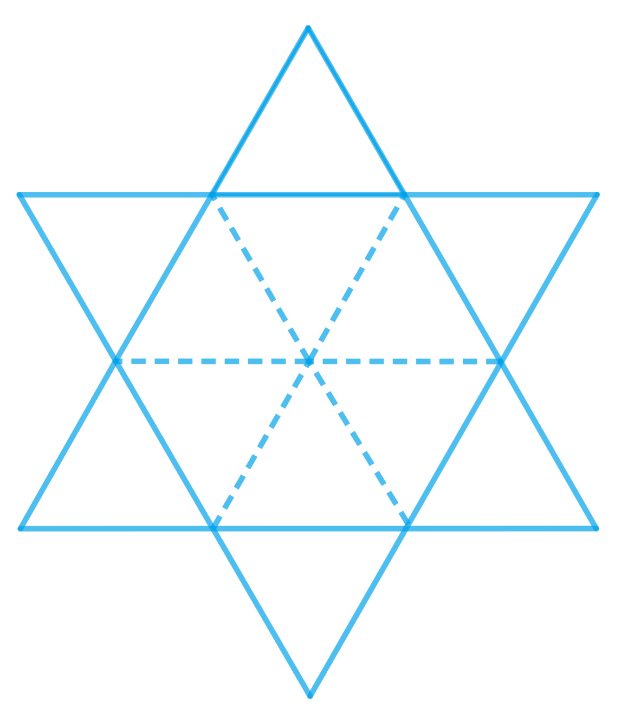Area of star-shaped rangoli

\begin{align}& = 12 \times \frac { \sqrt { 3 } } { 4 } \times ( 5 ) ^ { 2 } \\ & = 75 \sqrt { 3 } \end{align}

Number of equilateral triangles of 1 cm side that can be filled in this star-shaped rangoli

\begin{align} &= \left( \frac { 75 \sqrt { 3 } } { \frac { \sqrt { 3 } } { 4 } } \right) \\ &= 300 \end{align}

Therefore, star-shaped rangoli has more equilateral triangles in it.

Instant doubt clearing with Cuemath Advanced Math Program